2、继承的类和实现的接口

public class HashMap<K,V> extends AbstractMap<K,V>
implements Map<K,V>, Cloneable, Serializable {

3、HashMap中的成员变量、数据结构、常用方法

3.1、成员变量

/**
* Holds cached entrySet(). Note that AbstractMap fields are used
* for keySet() and values().
*/
transient Set<Map.Entry<K,V>> entrySet;

transient int size;

/**
* The next size value at which to resize (capacity * load factor).
*
* @serial
*/
// (The javadoc description is true upon serialization.
// Additionally, if the table array has not been allocated, this
// field holds the initial array capacity, or zero signifying
// DEFAULT_INITIAL_CAPACITY.)
int threshold;

/**
* The load factor for the hash table.
*
* @serial
*/

3.2、常量

/**
* The default initial capacity - MUST be a power of two.
*/
static final int DEFAULT_INITIAL_CAPACITY = 1 << 4; // aka 16

/**
* The maximum capacity, used if a higher value is implicitly specified
* by either of the constructors with arguments.
* MUST be a power of two <= 1<<30.
*/
static final int MAXIMUM_CAPACITY = 1 << 30;

/**
* The load factor used when none specified in constructor.
*/
static final float DEFAULT_LOAD_FACTOR = 0.75f;

/**
* The bin count threshold for using a tree rather than list for a
* bin.  Bins are converted to trees when adding an element to a
* bin with at least this many nodes. The value must be greater
* than 2 and should be at least 8 to mesh with assumptions in
* tree removal about conversion back to plain bins upon
* shrinkage.
*/
static final int TREEIFY_THRESHOLD = 8;

/**
* The bin count threshold for untreeifying a (split) bin during a
* resize operation. Should be less than TREEIFY_THRESHOLD, and at
* most 6 to mesh with shrinkage detection under removal.
*/
static final int UNTREEIFY_THRESHOLD = 6;

/**
* The smallest table capacity for which bins may be treeified.
* (Otherwise the table is resized if too many nodes in a bin.)
* Should be at least 4 * TREEIFY_THRESHOLD to avoid conflicts
* between resizing and treeification thresholds.
*/
static final int MIN_TREEIFY_CAPACITY = 64;

the bin。。 说一个 哈希表中的一个 常用术语：  bucket  桶。

bucket的英文解释：

Hash table lookup operations are often O(n/m) (where n is the number of objects in the table and m is the number of buckets), which is close to O(1), especially when the hash function has spread the hashed objects evenly through the hash table, and there are more hash buckets than objects to be stored.

• 要存数据时，第一次HASH到这里，在第一个BUCKET存放一个数据。
• 要存数据时，当第二次因某些原因HASH到这里时，在第二个BUCKET存放另一个数据。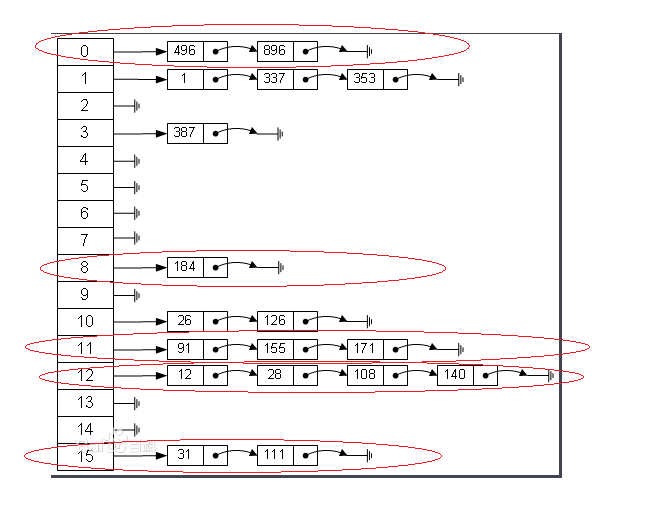ps；是否 达到  根据  size 去衡量的，而不是 非空桶去 衡量的，没有数据的是空桶比如 4 ，5，6，7。

3.3、数据结构

transient Node<K,V>[] table;

/**
* Holds cached entrySet(). Note that AbstractMap fields are used
* for keySet() and values().
*/

**
* Basic hash bin node, used for most entries.  (See below for
* TreeNode subclass, and in LinkedHashMap for its Entry subclass.)
*/
static class Node<K,V> implements Map.Entry<K,V> {
//哈希值，具体的这几个使用到哈希算法的集合中，哈希算法都重写了，有区别的
final int hash;
//key值，也就是你存储对象的别名吧。暂时这么理解，这个属于唯一不可重复的。
final K key;
//真正存你的数据的地方。
V value;
//下一个节点的引用，因为哈希表是一个数组+链表的组合。
Node<K,V> next;
// 构造方法。
Node(int hash, K key, V value, Node<K,V> next) {
this.hash = hash;
this.key = key;
this.value = value;
this.next = next;
}

（以前没注意，这次看了看类，发现里面的内部类好多啊！！！！！就写个我知道的吧，其他的真是用过但是没去仔细看过，等看过再添加一下）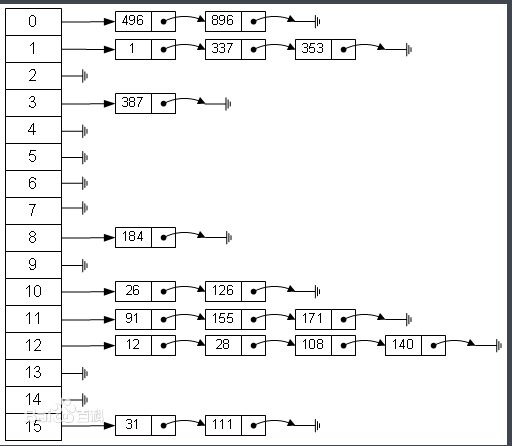3.4 常用方法

3.4.1 构造方法

3.4.1.1 无参构造方法

/**
* Constructs an empty <tt>HashMap</tt> with the default initial capacity
* (16) and the default load factor (0.75).
*/
public HashMap() {
this.loadFactor = DEFAULT_LOAD_FACTOR; // all other fields defaulted
}

3.4.1.2 带长度的构造方法

/**
* Constructs an empty <tt>HashMap</tt> with the specified initial
* capacity and the default load factor (0.75).
*
* @param  initialCapacity the initial capacity.
* @throws IllegalArgumentException if the initial capacity is negative.
*/
public HashMap(int initialCapacity) {
}

3.4.1.3 带长度，负载因子的构造方法

public HashMap(int initialCapacity, float loadFactor) {
if (initialCapacity < 0)
throw new IllegalArgumentException("Illegal initial capacity: " +
initialCapacity);
if (initialCapacity > MAXIMUM_CAPACITY)
initialCapacity = MAXIMUM_CAPACITY;
throw new IllegalArgumentException("Illegal load factor: " +
this.threshold = tableSizeFor(initialCapacity);
}

3.4.1.4 传入一个Map的实现子类的集合

public HashMap(Map<? extends K, ? extends V> m) {
putMapEntries(m, false);
}

这个是传入一个 map 的子类，然后转换成一个 HashMap， 我是没怎么用过。。

高能预警

3.4.2 put方法

/**
* Associates the specified value with the specified key in this map.
* If the map previously contained a mapping for the key, the old
* value is replaced.
*
* @param key key with which the specified value is to be associated
* @param value value to be associated with the specified key
* @return the previous value associated with <tt>key</tt>, or
*         <tt>null</tt> if there was no mapping for <tt>key</tt>.
*         (A <tt>null</tt> return can also indicate that the map
*         previously associated <tt>null</tt> with <tt>key</tt>.)
*/
public V put(K key, V value) {
return putVal(hash(key), key, value, false, true);
}

static final int hash(Object key) {
int h;
return (key == null) ? 0 : (h = key.hashCode()) ^ (h >>> 16);
}

public native int hashCode();

public int hashCode() {
int h = hash;
if (h == 0 && value.length > 0) {
char val[] = value;

for (int i = 0; i < value.length; i++) {
h = 31 * h + val[i];
}
hash = h;
}
return h;
}

public int hashCode() {
return Integer.hashCode(value);
}
public static int hashCode(int value) {
return value;
}

/**
*将上面的代码进行分步，更加便于理解
*
*/
int h=key.hashCode();
//这个用人话就是 h和h/2的16次方的数 进行 与运算
int hash=  h^h>>>16;
return hash;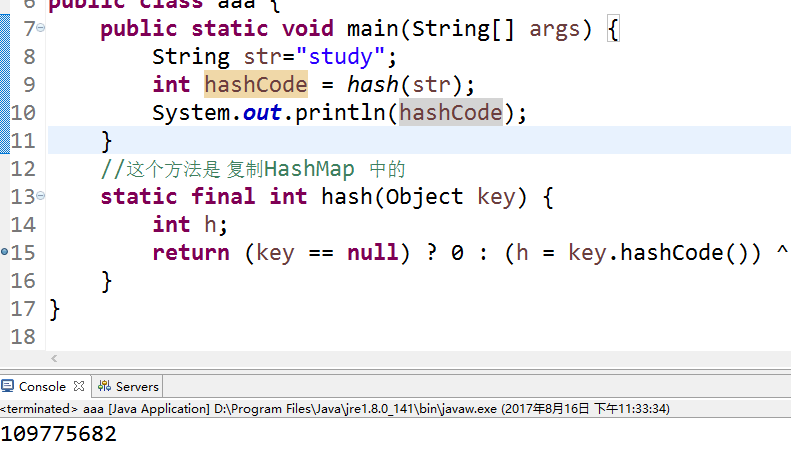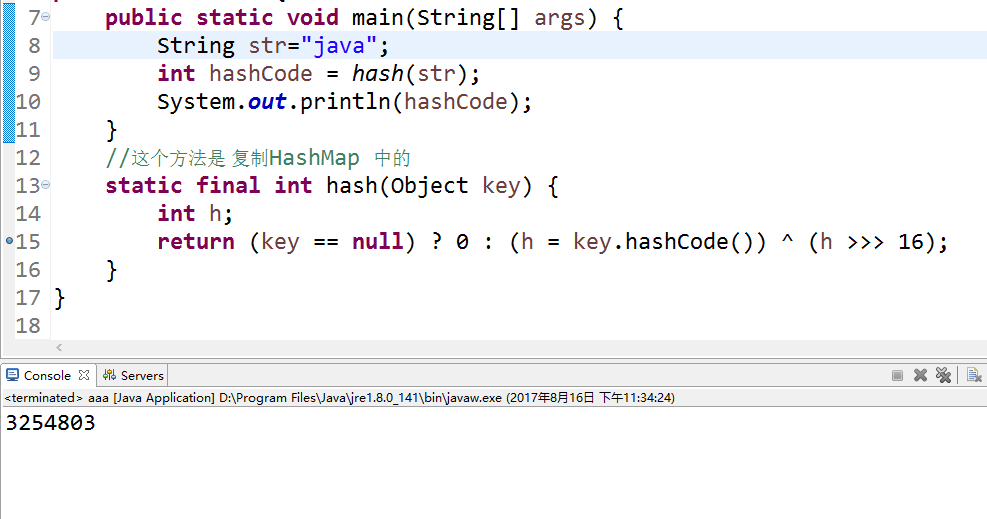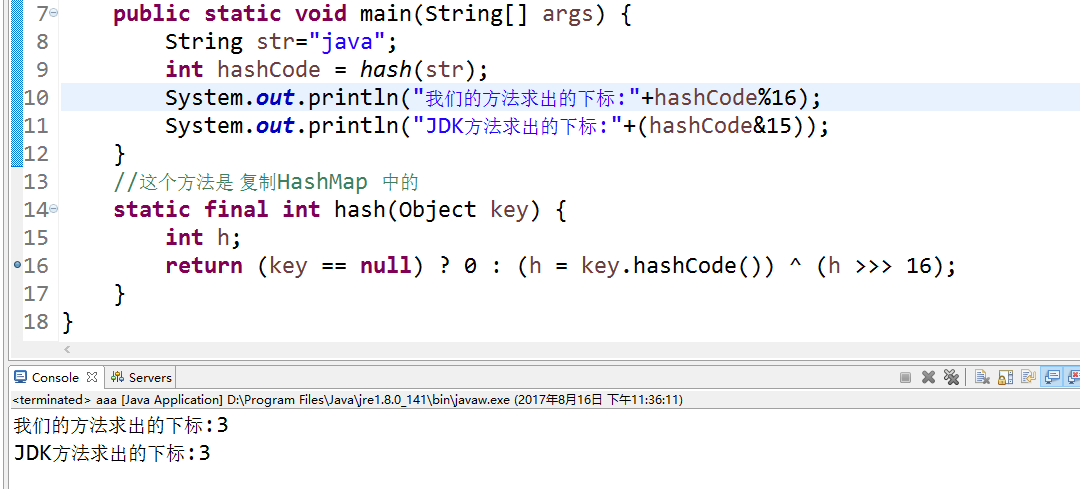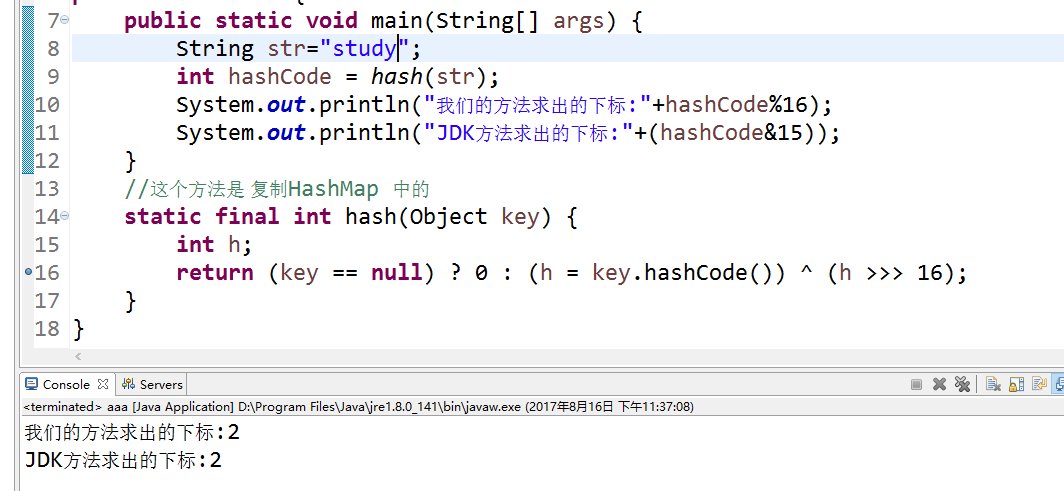细心的同学会发现，  这两个图的 11 行 hashCode&15

static final int hash(Object key) {   //jdk1.8 & jdk1.7
int h;
// h = key.hashCode() 为第一步 取hashCode值
// h ^ (h >>> 16)  为第二步 高位参与运算
return (key == null) ? 0 : (h = key.hashCode()) ^ (h >>> 16);
}

static int indexFor(int h, int length) {
//jdk1.7的源码，jdk1.8没有这个方法，但是实现原理一样的
// jdk1.8中是在put的时候，直接使用的 h&(length-1)的，而不是写了一个方法
return h & (length-1);  //第三步 取模运算
}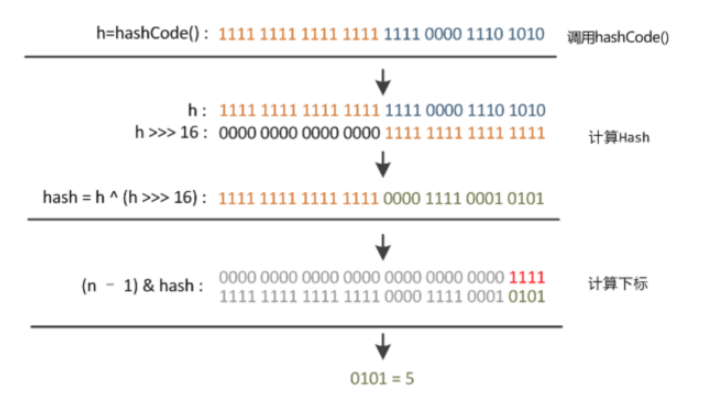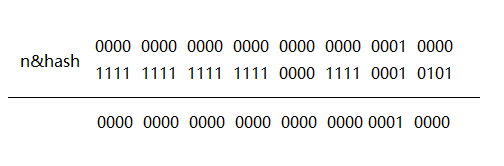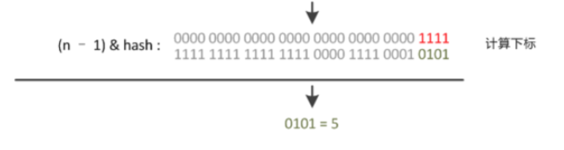/**
* Implements Map.put and related methods
*
* @param hash hash for key
* @param key the key
* @param value the value to put
* @param onlyIfAbsent if true, don't change existing value
* @param evict if false, the table is in creation mode.
* @return previous value, or null if none
*/
final V putVal(int hash, K key, V value, boolean onlyIfAbsent,
boolean evict) {
Node<K,V>[] tab; Node<K,V> p; int n, i;
//首先判断table 是不是空，或者是不是为null
if ((tab = table) == null || (n = tab.length) == 0)
//扩容，其实不明白为什么不初始化直接初始化好，而是用的时候才扩容。。。
n = (tab = resize()).length;
// 判断 得到的 下表位置是否存在数据
if ((p = tab[i = (n - 1) & hash]) == null)
//不存在就直接放入就行
tab[i] = newNode(hash, key, value, null);
else {
Node<K,V> e; K k;
// 如果已经存在数据了，就去遍历这个桶的 链表进行比较。

// 先判断 这个点的 hash 是否一样，如果一样比较key 的地址，最后使用equals方法比较
//注意几点：hash一样，key不一定一样（几率特小）
// key一样，hash肯定一样（计算方法一样，肯定一样）
// == 一样，表示是同一对象，肯定equals 一样。
// 这里面  有  短路  现象， 自查~
if (p.hash == hash &&
((k = p.key) == key || (key != null && key.equals(k))))
//如果符合条件，就得到这个 节点 的引用
e = p;
else if (p instanceof TreeNode)
//说实在，这个不是太明白为什么
e = ((TreeNode<K,V>)p).putTreeVal(this, tab, hash, key, value);
else {
//接下来是遍历链表查找，表中的 key是否已经存在，
for (int binCount = 0; ; ++binCount) {
//先指向下一个节点，如果是null，就将 node 放入到这里，
//第一个节点已经判断过了，这种方式类似do{}while()吧。
if ((e = p.next) == null) {
p.next = newNode(hash, key, value, null);
if (binCount >= TREEIFY_THRESHOLD - 1) // -1 for 1st
treeifyBin(tab, hash);
break;
}
// 判断条件同上
if (e.hash == hash &&
((k = e.key) == key || (key != null && key.equals(k))))
break;
p = e;
}
}
//如果 e 是存在的，也就是说，key值已经 存过了
// 这就是 为什么 HashMap为什么不能存重复key 的原因了！
if (e != null) { // existing mapping for key
//得到老的值
V oldValue = e.value;
if (!onlyIfAbsent || oldValue == null)
//新值直接覆盖
e.value = value;
//这个函数不知道。。暂时不管他吧
afterNodeAccess(e);
return oldValue;
}
}
++modCount;
if (++size > threshold)
resize();//扩容方法
afterNodeInsertion(evict);
return null;
}

3.2.3 resize

final Node<K,V>[] resize() {
//记录扩容前的Hash表
Node<K,V>[] oldTab = table;
//记录扩容前数组的长度
int oldCap = (oldTab == null) ? 0 : oldTab.length;
int oldThr = threshold;
int newCap, newThr = 0;
//这下面是判断 扩容前的长度是否是一个 异常的长度
if (oldCap > 0) {
//如果扩容前的长度超过了 规定的最大的长度
if (oldCap >= MAXIMUM_CAPACITY) {
threshold = Integer.MAX_VALUE;
return oldTab;
}
//扩容前的长度  *2 是否 超过规定的最大值（这扩容也是每次都是 2被，初始化 是 16，每次都是2倍，
// 扩容后还是2的 整数幂
else if ((newCap = oldCap << 1) < MAXIMUM_CAPACITY &&
oldCap >= DEFAULT_INITIAL_CAPACITY)
newThr = oldThr << 1; // double threshold

}
// 这一堆 判断 就不看了。。
else if (oldThr > 0) // initial capacity was placed in threshold
newCap = oldThr;
else {               // zero initial threshold signifies using defaults
newCap = DEFAULT_INITIAL_CAPACITY;
newThr = (int)(DEFAULT_LOAD_FACTOR * DEFAULT_INITIAL_CAPACITY);
}
if (newThr == 0) {
float ft = (float)newCap * loadFactor;
newThr = (newCap < MAXIMUM_CAPACITY && ft < (float)MAXIMUM_CAPACITY ?
(int)ft : Integer.MAX_VALUE);
}
threshold = newThr;
@SuppressWarnings({"rawtypes","unchecked"})
//初始化一个table
Node<K,V>[] newTab = (Node<K,V>[])new Node[newCap];
table = newTab;
//判断 扩容前的表是不是一个null 的，如果是null，就不用数据复制了。
if (oldTab != null) {
for (int j = 0; j < oldCap; ++j) {
Node<K,V> e;
//如果 当前桶 不为空
if ((e = oldTab[j]) != null) {
//将桶制空，当所有桶都空，方便gc回收
oldTab[j] = null;
if (e.next == null)
//再次用hash值进行求一个 index
newTab[e.hash & (newCap - 1)] = e;
else if (e instanceof TreeNode)
((TreeNode<K,V>)e).split(this, newTab, j, oldCap);
else { // preserve order
//如果当前桶 有一个链表
Node<K,V> loHead = null, loTail = null;
Node<K,V> hiHead = null, hiTail = null;
Node<K,V> next;

do {
next = e.next;
//这里为什么这么处理???
if ((e.hash & oldCap) == 0) {
//这个判断如果 是不是 空，不是空，就接着组成一个链表
if (loTail == null)
else
loTail.next = e;
loTail = e;
}
else {
if (hiTail == null)
else
hiTail.next = e;
hiTail = e;
}
} while ((e = next) != null);
//把分好类的 放入到 新的table 数组中
//我们发现 如果之前的 hash&length ==0 就放入newtable[j]
if (loTail != null) {
loTail.next = null;
}
//我们发现 如果之前的 hash&length !=0 就放入newtable[j+oldCap]
if (hiTail != null) {
hiTail.next = null;
newTab[j + oldCap] = hiHead;
}
}
}
}
}
return newTab;
}

resize 也是一个大工程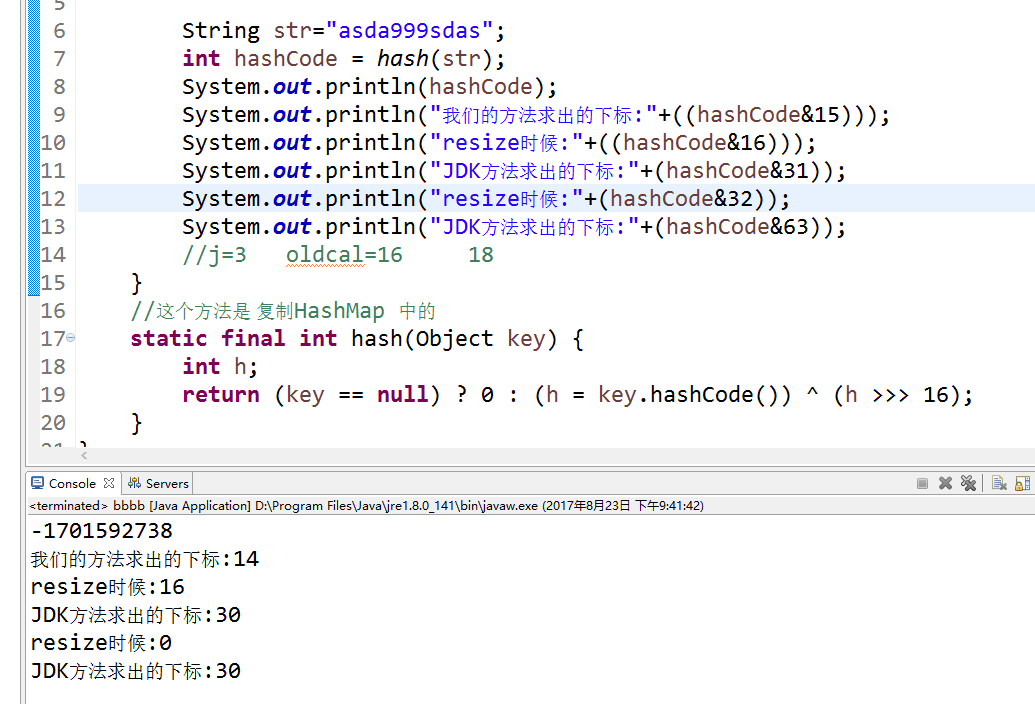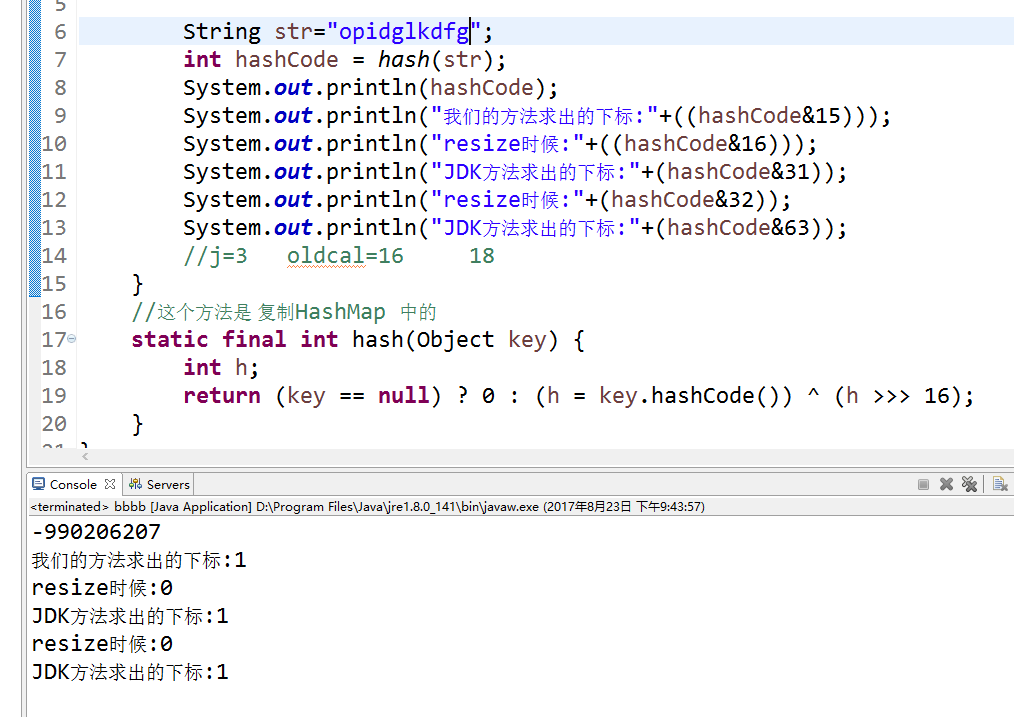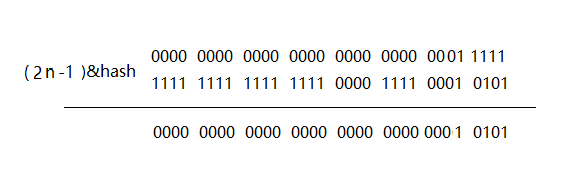3.2.4 remove

put方法就是核心，讲解完了之后，后面的应该都不是问题：

public V remove(Object key) {
Node<K,V> e;
return (e = removeNode(hash(key), key, null, false, true)) == null ?
null : e.value;
}

remove 内层调用方法

final Node<K,V> removeNode(int hash, Object key, Object value,
boolean matchValue, boolean movable) {
Node<K,V>[] tab; Node<K,V> p; int n, index;
//判断标是不是空， 同时判断 表长是不是 大于 0， 还有 直接使用 hash算法求得桶的第一个是不是null
if ((tab = table) != null && (n = tab.length) > 0 &&
(p = tab[index = (n - 1) & hash]) != null) {
Node<K,V> node = null, e; K k; V v;
//先判断第一个点，判断方法相同
if (p.hash == hash &&
((k = p.key) == key || (key != null && key.equals(k))))
node = p;
else if ((e = p.next) != null) {
//不知道什么意思
if (p instanceof TreeNode)
node = ((TreeNode<K,V>)p).getTreeNode(hash, key);
else {
//从第二个点开始 遍历 判断是否存在 相同key
do {
if (e.hash == hash &&
((k = e.key) == key ||
(key != null && key.equals(k)))) {
node = e;
break;
}
p = e;
} while ((e = e.next) != null);
}
}
//如果找到了 这个节点（不是null），就进行移除。
if (node != null && (!matchValue || (v = node.value) == value ||
(value != null && value.equals(v)))) {
if (node instanceof TreeNode)
((TreeNode<K,V>)node).removeTreeNode(this, tab, movable);
else if (node == p)
tab[index] = node.next;
else
p.next = node.next;
++modCount;
--size;
afterNodeRemoval(node);
return node;
}
}
return null;
}

3.2.5 get

public V get(Object key) {
Node<K,V> e;
return (e = getNode(hash(key), key)) == null ? null : e.value;
}

final Node<K,V> getNode(int hash, Object key) {
Node<K,V>[] tab; Node<K,V> first, e; int n; K k;
if ((tab = table) != null && (n = tab.length) > 0 &&
(first = tab[(n - 1) & hash]) != null) {
if (first.hash == hash && // always check first node
((k = first.key) == key || (key != null && key.equals(k))))
return first;
if ((e = first.next) != null) {
if (first instanceof TreeNode)
return ((TreeNode<K,V>)first).getTreeNode(hash, key);
do {
if (e.hash == hash &&
((k = e.key) == key || (key != null && key.equals(k))))
return e;
} while ((e = e.next) != null);
}
}
return null;
}

get 方法是同样的套路，都是 遍历查找，用的判断条件也都一样，hash，下表的求法都大同小异，偷个懒，这些都不用去解释了吧。

3.5 其他方法总结

 是否包含对应的key public boolean containsKey(Object key) 是否包含对应的value public boolean containsValue(Object value) 放入一个map的子类 public void putAll(Map m) 返回size public int size() 返回是否为空 public boolean isEmpty()

总结：

HashMap 为什么不能重复？ （put 方法第一行就处理了，是null得 hash值是 0 ）

-----------------------------------------------------------------------------

jsonObject 解析map 字段问题

2015/08/02
547
5
ArrayList 和 HaspMap 链式添加的实现

_魔术师_
2013/11/13
472
0
Java 容器 & 泛型：五、HashMap 和 TreeMap的自白

Writer：BYSocket（泥沙砖瓦浆木匠） 微博：BYSocket 豆瓣：BYSocket Java 容器的文章这次应该是最后一篇了：Java 容器 系列。 今天泥瓦匠聊下 Maps。 一、Map回顾 Map，又称映射表，是将键映...

2015/05/05
1K
0
HaspMap 多线程下 resize 死循环

HashMap在多线程下面出现死循环，这种情况有点难模拟，千万别在生成环境出现，一旦出现那就等着哭吧。 废话不多说不知道hashmap 如何扩容的先看下源码可能好理解点。 void resize(int newCap...

smallsun512
2013/12/26
508
0
Java中HashMap遍历

2016/08/10
0
0

CREATE_17

5
0
CSS盒子模型

CSS盒子模型 组成： content --> padding --> border --> margin 像现实生活中的快递： 物品 --> 填充物 --> 包装盒 --> 盒子与盒子之间的间距 content ：width、height组成的 内容区域 padd......

studywin

7
0

locbytes

8
0
jquery 添加和删除节点

8
0

ZeroBit

10
0